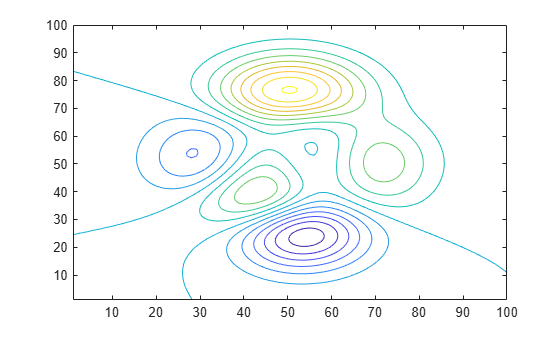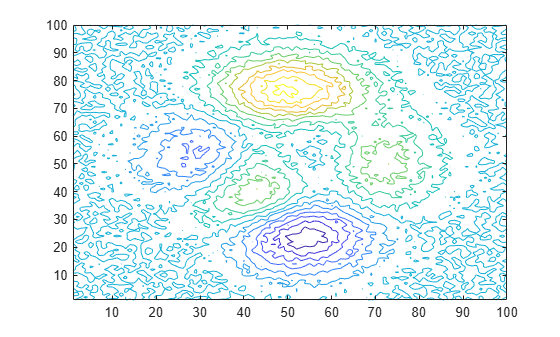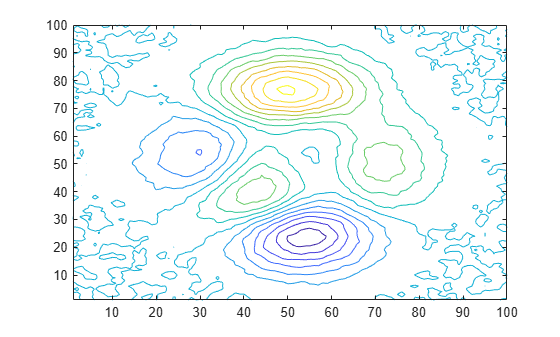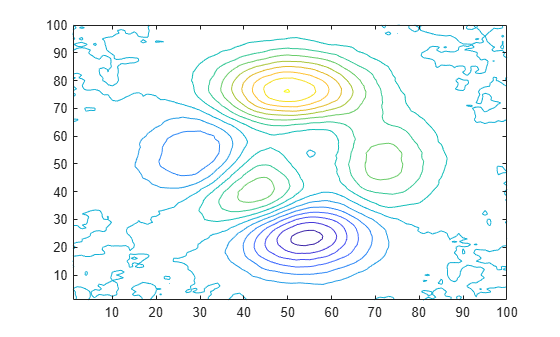# Smooth Data with Convolution

You can use convolution to smooth 2-D data that contains high-frequency components.

Create 2-D data using the `peaks` function, and plot the data at various contour levels.

```Z = peaks(100); levels = -7:1:10; contour(Z,levels)```Inject random noise into the data and plot the noisy contours.

```Znoise = Z + rand(100) - 0.5; contour(Znoise,levels)```The `conv2` function in MATLAB® convolves 2-D data with a specified kernel whose elements define how to remove or enhance features of the original data. Kernels do not have to be the same size as the input data. Small-sized kernels can be sufficient to smooth data containing only a few frequency components. Larger sized kernels can provide more precision for tuning frequency response, resulting in smoother output.

Define a 3-by-3 kernel `K` and use `conv2` to smooth the noisy data in `Znoise`. Plot the smoothed contours. The `'same'` option in `conv2` makes the output the same size as the input.

```K = (1/9)*ones(3); Zsmooth1 = conv2(Znoise,K,'same'); contour(Zsmooth1, levels)```Smooth the noisy data with a 5-by-5 kernel, and plot the new contours.

```K = (1/25)*ones(5); Zsmooth2 = conv2(Znoise,K,'same'); contour(Zsmooth2,levels)```